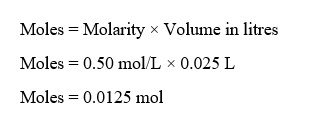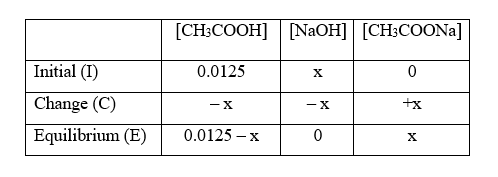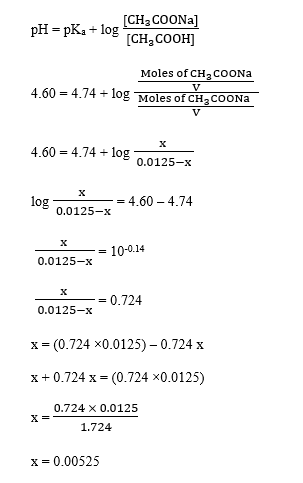# what volume of 0.30 M sodium hydroxide must be added to 25.0 ml of 0.500 M acetic acid to produce a solution with a pH of 4.60?

Question
2 views

what volume of 0.30 M sodium hydroxide must be added to 25.0 ml of 0.500 M acetic acid to produce a solution with a pH of 4.60?

check_circle

Step 1

Given,

Molarity of sodium hydroxide, NaOH = 0.30 M = 0.30 mol/L

Molarity of acetic acid, CH3COOH = 0.50 M = 0.50 mol/L

Volume of acetic acid = 25.0 mL = 0.025 L   (1 mL = 0.001 L)

pH of the solution = 4.60

Moles of CH3COOH can be calculated as :Step 2

Since the pH is 4.60 which is less than 7, so the solution is acidic, that means all of base, NaOH is consumed in the reaction.

Let the moles of NaOH be x.

The reaction involved is :

CH3COOH (aq) + NaOH (aq) → CH3COONa (aq) + H2O (l)

The ICE table for this reaction can be written as :Step 3

pKa of acetic acid = 4.74

Let V be the total volume of the solution.

Using the Henderson Hasselbalch equation, we get,...

### Want to see the full answer?

See Solution

#### Want to see this answer and more?

Solutions are written by subject experts who are available 24/7. Questions are typically answered within 1 hour.*

See Solution
*Response times may vary by subject and question.
Tagged in